### Thermistors

Basically thermistor is a contraction of the word 'thermal resistors', The resistors depending on temperature are thermal resistors. Thus resistance thermometers are also thermistors having positive -temperature coefficients. But generally the resistors having negative temperature coefficients (NTC) are called thermistors. The resistance of a thermistor decreases as temperature increases. The NTC of thermistors can be as large as a few percent per degree celsius change in temperature. Thus the thermistors are very sensitive and can detect very small changes in temperature too.

Construction of Thermistor:

• Thermistors are composed of a sintered mixture of metallic oxides, such as manganese, nickel, cobalt, copper, iron, and uranium. Their resistances at ambient temperature may range from 100 n to 100 ill. Thermistors are available in a wide variety of shapes and sizes as shown in the Fig. Smallest in size are the beads with a diameter of 0.15 mm to 1.25 mm. Beads may be sealed in the tips of solid glass rods to form probes. Disks and washers are made by pressing thermistor material under high pressure into Hat cylindrical shapes. Washers can be placed in series or in parallel to increase power dissipation rating.

• Thermistors are well suited for precision temperature measurement, temperature control, and temperature compensation, because of their. very large change in resistance with temperature. They are widely used for measurements in the temperature range -1000 C to +2000 C. The measurement of the change in resistance with temperature is carried out with a Wheatstone bridge.

Inductive Transducer

Inductive transducers work on the principle of inductance change due to any appreciable change in the quantity to be measured i.e. measured. For example, LVDT, a kind of inductive transducers, measures displacement in terms of voltage difference between its two secondary voltages. Secondary voltages are nothing but the result of induction due to the flux change in the secondary coil with the displacement of the iron bar. Anyway LVDT is discussed here briefly to explain the principle of inductive transducer. LVDT will be explained in other article in more detail. For the time being let‟s focus on basic introduction of inductive transducers. Now first our motive is to find how the inductive transducers can be made to work. This can be done by changing the flux with the help of measured and this changing flux obviously changes the inductance and this inductance change can be calibrated in terms of measured. Hence inductive transducers use one of the following principles for its working.

1. Change of self inductance

2. Change of mutual inductance

3. Production of eddy current

Change of Self Inductance of Inductive Transducer

We know very well that self inductance of a coil is given by

Where, N = number of turns. R = reluctance of the magnetic circuit.

Also we know that reluctance R is given by

where μ = effective permeability of the medium in and around the coil.

Where, G = A/l and called geometric form factor. A = area of cross-section of coil. l = length of the coil. So, we can vary self inductance by

1. Change in number of turns, N,

2. Changing geometric configuration, G,

3. Changing permeability

For the sake of understanding we can say that if the displacement is to be measured by the inductive transducers, it should change any of the above parameter for causing in the change in self inductance

Change of Mutual Inductance of Inductive Transducer Here transducers

• which work on change of mutual inductance principle, use multiple coils. We use two coils here for the sake of understanding. Both coils have their self inductance as well. So let‟s denote their self inductance by L1 and L2. Mutual inductance between these two coils is given by

• Thus mutual inductance can be changed by varying self inductance or by varying coefficient of coupling, K. The methods of changing self inductance we already discussed. Now the coefficient of coupling depends on the distance and orientation between two coils. Thus for the measurement of displacement we can fix one coil and make other movable which moves with the source whose displacement is to be measured. With the change in distance in displacement coefficient of coupling changes and it causes the change in mutual inductance. This change in mutual inductance can be calibrated with the displacement and measurement can be done.

Production of Eddy Current of Inductive Transducer

• We know that when a conducting plate is placed near a coil carrying alternating current, a circulating current is induced in the plate called “EDDY CURRENT”. This principle is used in such type of inductive transducers. Actually what happens? When a coil is placed near to coil carrying alternating current, a circulating current is induced in it which in turn produces its own flux which try to reduce the flux of the coil carrying the current and hence inductance of the coil changes. Nearer the plate is to the coil, higher will be eddy current and higher is the reduction in inductance and vice versa.

• Thus inductance of coil varied with the variation of distance between coil and plate. Thus the movement of the plate can be calibrated in terms of inductance change to measure the quantity like displacement.

## Linear variable differential transformer (LVDT)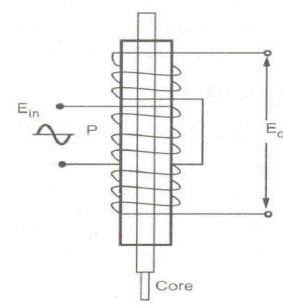• When an externally applied force moves the core to the left-hand position, more magnetic flux links the left-hand coil than the right hand coil. The emf induced in the left-hand coil, ES], is therefore larger than the induced emf of the right-hand [oil, Es2' The magnitude of the output voltage is then equal to the difference between the two secondary voltages and it is in phase with the voltage of the left-hand coil.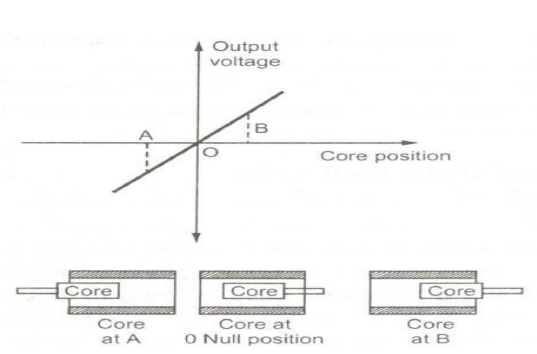Construction of LVDT:

• Main Features of Construction are as,

• The transformer consists of a primary winding P and two secondary winding S1 and S2 wound on a cylindrical former(which is hollow in nature and will contain core).

• Both the secondary windings have equal number of turns and are identically placed on the either side of primary winding .The primary winding is connected to an AC source which produces a flux in the air gap and voltages are induced in secondary windings.  A movable soft iron core is placed inside the former and displacement to be measured is connected to the iron core.

• The iron core is generally of high permeability which helps in reducing harmonics and high sensitivity of LVDT.  The LVDT is placed inside a stainless steel housing because it will provide electrostatic and electromagnetic shielding. Both the secondary windings are connected in such a way that the resulting output is the difference of the voltages of two windings.

Principle of Operation and Working:

• As the primary is connected to an AC source so alternating current and voltages are produced in the secondary of the LVDT. The output in secondary S1 is e1 and in the secondary S2 is e2. So

• the differential output is, eout = e1 - e2 This equation explains the principle of Operation of LVDT.

• Now three cases arise according to the locations of core which explains the working of LVDT are discussed below as,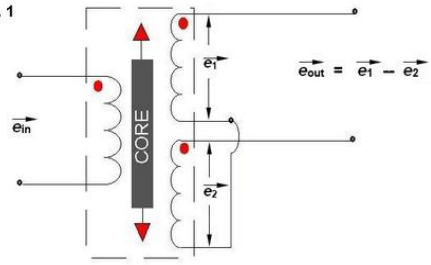• Case I: When the core is at null position (for no displacement) When the core is at null position then the flux linking with both the secondary windings is equal so the induced emf is equal in both the windings. So for no displacement the value of output eout is zero as e1 and e2 both are equal. So it shows that no displacement took place.

• Case II: When the core is moved to the upward of null position (For displacement to the upward of reference point) In this case the flux linking with secondary winding S1 is more as compared to flux linking with S2. Due to this e1 will be more as that of e2. Due to this output voltage eout is positive.

• CaseIII: When the core is moved to the downward of Null position (for displacement to the downward of reference point) In this case magnitude of e2 will be more as that of e1. Due to this output eout will be negative and shows the output to downward of reference point

• Output VS Core Displacement A linear curve shows that output voltage varies linearly with displacement of core.

Some important points about magnitude and sign of voltage induced in LVDT

1. The amount of change in voltage either negative or positive is proportional to the amount of movement of the core and indicates the amount of linear motion.

2. By noting the output voltage increasing or decreasing the direction of motion can be determined

3. The output voltage of an LVDT is a linear function of core displacement .

• High Range- The LVDTs have a very high range for measurement of displacement.they can used for measurement of displacements ranging from 1.25mm to 250mm

• No Frictional Losses- As the core moves inside a hollow former so there is no loss of displacement input as frictional loss so it makes LVDT as very accurate device.  High Input and High Sensitivity - The output of LVDT is so high that it doesn‟t need any amplification.the transducer possesses a high sensitivity which is typically about 40V/mm.

• Low Hysteresis- LVDTs show a low hysteresis and hence repeatability is excellent under all conditions

• Low Power Consumption- The power is about 1W which is very low as compared to other transducers.

• Direct Conversion to Electrical Signals- They convert the linear displacement to electrical voltage which are easy to process

• LVDT is sensitive to stray magnetic fields so they always require a setup to protect them from stray magnetic fields.

• They are affected by vibrations and temperature.

• It is concluded that they are advantageous as compared than any other inductive transducers.

Applications of LVDT

• They are used in applications where displacements ranging from fraction of mm to few cm are to be measured. The LVDT acting as a primary Transducer converts the displacement to electrical signal directly.

• They can also acts as the secondary transducers. E.g. the Bourbon tube which acts as a primary transducer and converts pressure into linear displacement.then LVDT converts this displacement into electrical signal which after calibration gives the ideas of the pressure of fluid.

Capacitive Transducers

• A capacitor consists of two conductors (plates) that are electrically isolated from one another by a nonconductor (dielectric). When the two conductors are at different potentials (voltages), the system is capable of storing an electric charge. The storage capability of a capacitor is measured in farads.The principle of operation of capacitive transducers is based upon the equation for capacitance of a parallel plate capacitor as shown in Fig.
Capacitance,

• Where, A = Overlapping area of plates;m2 ,
​​​​​​                 d = Distance between two plates; m,  = Permittivity (dielectric constant); F/m.

• Parallel Plate Capacitor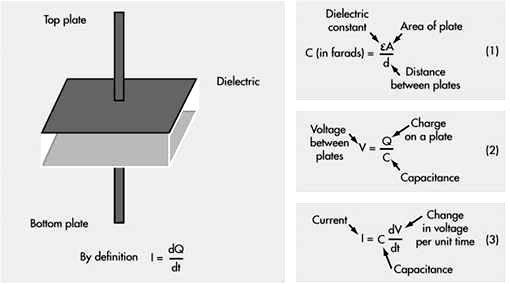• The capacitance is measured with a bridge circuit. The output impedance Z of a capacitive transducer is:

• Z = 1/2πfC
Where: Z = Impedance
f = frequency, 50 Hz.
C = capacitance

• In general, the output impedance of a capacitive transducer is high. This fact calls for a careful design of the output circuitry. The capacitive transducers work on the principle of change in capacitance  of  the  capacitor.  This  change  in  capacitance  could  be  caused  by  change  in overlapping area A of the plates, change in the distance d between the plates and change in dielectric constant

• In  most  of  the  cases  the  above  changes  are  caused  by  the  physical  variables,  such  as, displacement,  force  or  pressure.  Variation  in  capacitance  is  also  there  when  the  dielectric medium between the plates changes, as in the case of measurement of liquid or gas levels.Therefore,  the  capacitive  transducers  are  commonly  used  for  measurement  of  linear displacement, by employing the following effects as shown in Fig a and fig b.

• Change in capacitance due to change in overlapping area of plates.

• Change in capacitance due to change in distance between the two plates.

• Change in capacitance due to change in dielectric between the two plates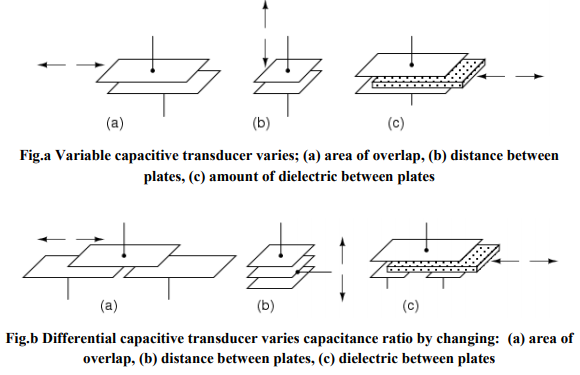• As may be seen in Fig b, all of the differential devices have three wire connections rather than two: one wire for each of the end plates and one for the common plate. As the capacitance between one of the endplates and the common plate changes, the capacitance between the other end plate and the common plate also changes in the opposite direction.

1. Transducers Using Change in Area of Plates
• Examining the equation for capacitance, it is found that the capacitance is directly proportional to the area, A of the plates. Thus, the capacitance changes linearly with change in area of plates. Hence  this  type  of  capacitive  transducer  is  useful  for  measurement  of  moderate  to  large displacements, say from 1 mm to several cm. The area changes linearly with displacement and also the capacitance.For a parallel plate capacitor, the capacitance is:

• Where,  l = length of overlapping part of plates; m, and

• w = width of overlapping part of plates; m.

Sensitivity,

• The sensitivity is constant and therefore there is a linear relationship between capacitance and displacement.This type of a capacitive transducer is suitable for measurement of linear displacement ranging from 1 to 10 cm. The accuracy is as high as 0.005%.

1. Transducers Using Change in Distance between Plates
• Fig. shows the basic form of a capacitive transducer employing change in distance between  the  two  plates  to  cause  the  change  in  capacitance.  One  plate  is  fixed  and  the displacement  to  be  measured  is  applied  to  the  other  plate  which  is  movable.  Since,  the capacitance,  C,  varies  inversely  as  the  distance  d,  between  the  plates  the  response  of  this transducer is not linear. Thus this transducer is useful only for measurement of extremely small displacements.

Sensitivity,

• Thus the sensitivity of this type of transducer is not constant but varies over the range of the transducer. The relationship between variations of capacitance with variation of distance between plates is hyperbolic and is only approximately linear over a small range of displacement. The linearity can be closely approximated by use of a piece of dielectric material like mica having a high dielectric constant, such as, a thin piece of mica.

1. Transducers Using Change in dielectric constant between Plates:
• If the area (A) of and the distance (d) between the plates  of a capacitor remain constant, capacitance will vary only as a function of the dielectric constant  of the substance filling the gap between the plates. If the space between the plates of a capacitor is filled with an insulator, the capacitance of the capacitor will change compared to the situation in which there is vacuum between the plates. The change in the capacitance is caused by a change in the electric field between the plates.

• The value of dielectric constant is initially set by design in the choice of dielectric material used to make the capacitor. Many factors will cause the  to change, and this change in will vary for different materials.  The  major  factors  that  will  cause  a  change  in   are  moisture,  voltage,  frequency,  and temperature. The dielectric constant of a process material can change due to variations in temperature, moisture,  humidity,  material  bulk  density,  and  particle  size  etc. The   in  the  basic  formula  is  the effective dielectric constant of the total space between the electrodes. This space may consist of the dielectric material, air, and even moisture, if present. The figure  shows how in a capacitor the position of the dielectric is varied to vary the capacitance. Physical variables, such as displacement, force or pressure can cause the movement of dielectric material in the capacitor plates, resulting in changes in the effective dielectric constant, which in turn will change the capacitance.

• Fig. Change in capacitance due to movement of dielectric between plates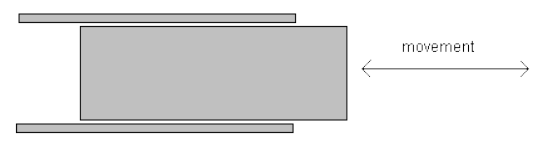• The major advantages of capacitive transducers are that they require extremely small forces to operate them and hence are very useful for use in small systems. They are extremely sensitive and require small power to operate them. Owing to their good frequency response they are very useful for dynamic studies.The disadvantages of capacitive transducers include their non-linear behavior on account of edge effects and the effects of stray capacitances especially when the transducers have a low value of capacitance. Therefore guard rings must be used to eliminate this effect. The metallic parts of the capacitive transducers must be insulated from each other. In order to reduce the effects of stray capacitances, the frames must be earthed.Capacitive transducers can be used for measurement of both linear and angular displacements.The capacitive transducers are highly sensitive and can be used for measurement of extremely hand, they can be used for measurement of large displacements up to about 30 m as in aeroplane altimeters. The change in area method is used for measurement of displacements ranging from 10 to 100 mm. Capacitive transducers can be used for the measurement of force and pressure. The force and pressure to be measured are first converted to displacement which causes a change of capacitance. Capacitive transducers can also be used directly as pressure transducers in all those cases where the dielectric constant of a medium changes with pressure. They can be used for measurement of humidity in gases and moisture content in soil / food products etc.

## Thermocouples

• Basically thermocouple consists of two different metals which are placed in contact with each other as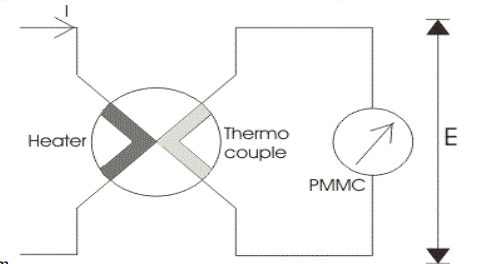• shown in the diagram.First part is called the heater element because when the current will flow through this, a heat is produced and thus the temperature will increase at the junction. At this junction an emf is produced which is approximately proportional to the temperature difference of hot and cold junctions.

• The emf produced is a DC voltage which is directly proportional to the root mean square value of electric current. A permanent magnet moving coil instrument is connected with the second part to read the current passing through the heater. One question must arise in our mind that why we have used only a permanent magnet coil instrument? Answer to this question is very easy because PMMC instrument has greater accuracy and sensitivity towards the measurement of DC value. The thermocouple type instruments employ thermocouple in their construction. Thermocouple type instruments can be used for both ac and DC applications. Also thermocouple type of instruments has greater accuracy in measuring the current and voltages at very high frequency accurately.

• Now we will look how the temperature difference is mathematically related to generated emf at the junction in thermocouple type of instruments. Let us consider the temperature of the heater element be Ta and the temperature of cold metal be Tb. Now it is found that the generated emf at the junction is related to temperature difference as:

• Where a and b are constant whose values completely depends upon the type of metal we are using. The

• above equation represents parabolic function. The approximated value of a is from 40 to 50 micro volts or

• more per degree Celsius rise in temperature and value of constant b is very small and can be neglected if the air gap field of permanent magnet moving coil is uniform. Thus we can approximate the above temperature emf relation as e = a(Ta - Tb), here we have assume b = 0. The current flowing through the heater coil produces heat as I2R where I is the root mean square value of current, if we assume the temperature of the cold junction is maintained at room temperature then the rise in the temperature of the hot junction will be equal to temperature rise at the junction. Hence we can write (Ta-Tb)is directly proportional to I2R or we can say (Ta - Tb) = kI2R. Now the deflection angle x in moving coil instrument is equal to; x = Ke or x = K[a(Ta - Tb)] hence we can write k.K.a.I2R = k1I2, where k1 is some constant.From the above equation we see that the instrument shows the square law response.

Construction of Thermocouple Type Instrument:

Now let us look at the construction of Thermocouple type Instruments. Broadly speaking the thermocouple type of instruments consists of two major parts which are written below:

1. Thermoelectric elements: The thermocouple type of instruments consists of thermoelectric elements which can be of four types:

1. Contact Type: It has a separate heater which is shown in the diagram.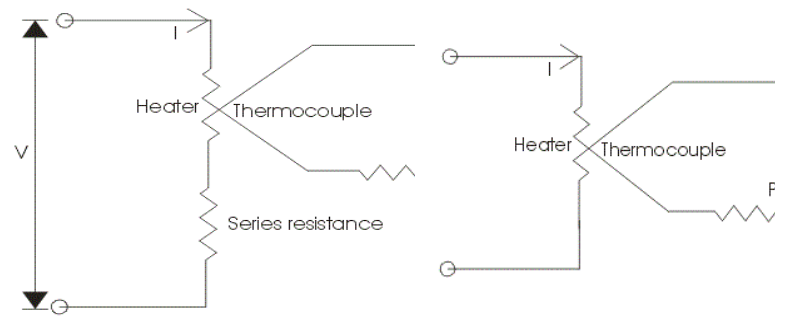• The action of thermocouple type instruments can be explained briefly as,

• At the junction the electrical energy is being converted to thermal energy in the heater element. A portion of the heat is transferred to the hot junction while most of the heat energy is dissipated away.

• The heat energy which is transferred to the hot junction is again converted to electrical due to Seebeck effect. Only a portion of electrical energy is converted into mechanical energy which is used to produce a deflecting torque. The overall efficiency of the system is low thus the instrument consumes high power. So there is a requirement for a highly accurate and sensitive DC instrument.

1. Non Contact Type: In non contact type there is insulation between the heating element and the thermocouple i.e. there is no direct contact between two. Due to this these instruments are not as sensitive as the contact type.

2. Vacuum Thermo-elements: These types of instruments are mostly employed for the measurement of electric current at very high frequency of the order of 100 Mega hertz or more as these instruments retain their accuracy even at such high frequency.

3. Bridge Type: These bridges are manufactured on the ac ratings usually from 100 mili amperes to 1 amperes. In this two thermocouple are connected to form a bridge which is shown in the figure given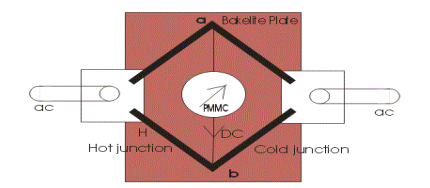below:

There is no requirement of heating element, the electric current which directly passes through the thermocouple raises the temperature which is directly proportional to the I2R losses. The bridge works in a balanced condition at which there will be no current in the arm ab. The connected meter will show the potential difference between the junctions a and b.